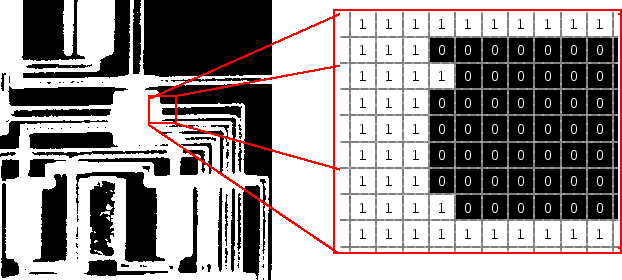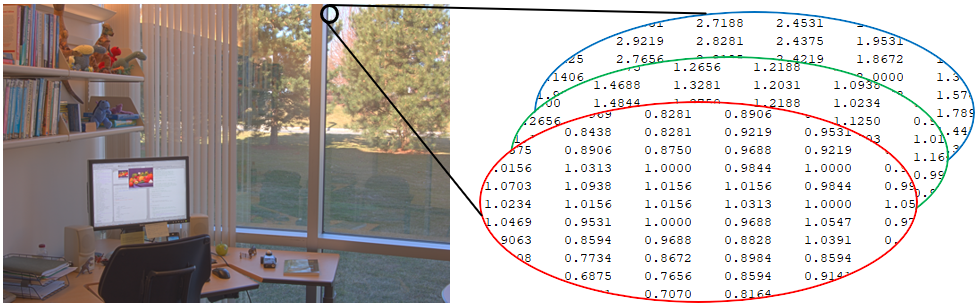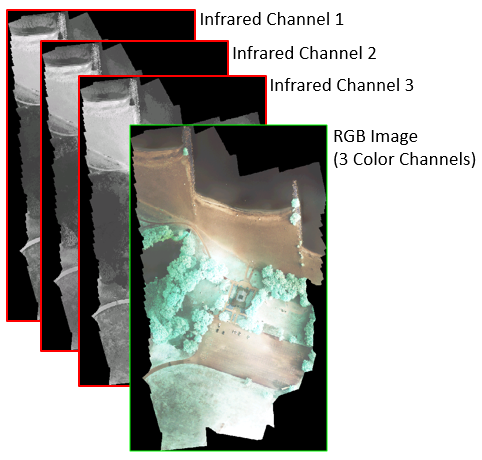## Image Types in the Toolbox

The Image Processing Toolbox™ software defines several fundamental types of images, summarized in the table. These image types determine the way MATLAB® interprets array elements as pixel intensity values.

All images in Image Processing Toolbox are assumed to have nonsparse values. Numeric and logical images are expected assumed to be real-valued unless otherwise specified.

Image Type

Interpretation

Image data are stored as an m-by-n logical matrix in which values of 0 and 1 are interpreted as black and white, respectively. Some toolbox functions can also interpret an m-by-n numeric matrix as a binary image, where values of 0 are black and all nonzero values are white.

Image data are stored as an m-by-n numeric matrix whose elements are direct indices into a color map. Each row of the color map specifies the red, green, and blue components of a single color.

• For `single` or `double` arrays, integer values range from [1, p].

• For `logical`, `uint8`, or `uint16` arrays, values range from [0, p-1].

The color map is a c-by-3 array of class `double`.

(Also known as intensity images)

Image data are stored as an m-by-n numeric matrix whose elements specify intensity values. The smallest value indicates black and the largest value indicates white.

• For `single` or `double` arrays, values range from [0, 1].

• For `uint8` arrays, values range from [0, 255].

• For `uint16`, values range from [0, 65535].

• For `int16`, values range from [-32768, 32767].

(Commonly referred to as RGB images)

Image data are stored as an m-by-n-by-3 numeric array whose elements specify the intensity values of one of the three color channels. For RGB images, the three channels represent the red, green, and blue signals of the image.

• For `single` or `double` arrays, RGB values range from [0, 1].

• For `uint8` arrays, RGB values range from [0, 255].

• For `uint16`, RGB values range from [0, 65535].

There are other models, called color spaces, that describe colors using three color channels. For these color spaces, the range of each data type may differ from the range allowed by images in the RGB color space. For example, pixel values in the L*a*b* color space of data type `double` can be negative or greater than 1. For more information, see Understanding Color Spaces and Color Space Conversion.

High Dynamic Range (HDR) ImagesHDR images are stored as an m-by-n numeric matrix or m-by-n-by-3 numeric array, similar to grayscale or RGB images, respectively. HDR images have data type `single` or `double` but data values are not limited to the range [0, 1] and can contain `Inf` values. For more information, see Work with High Dynamic Range Images.
Multispectral and Hyperspectral Images

Image data are stored as an m-by-n-by-c numeric array, where c is the number of color channels.

Label Images

Image data are stored as an m-by-n categorical matrix or numeric matrix of nonnegative integers.

### Binary Images

In a binary image, each pixel has one of only two discrete values: 1 or 0. Most functions in the toolbox interpret pixels with value 1 as belonging to a region of interest, and pixels with value 0 as the background. Binary images are frequently used in conjunction with other image types to indicate which portions of the image to process.

The figure shows a binary image with a close-up view of some of the pixel values.### Indexed Images

An indexed image consists of an image matrix and a color map.

A color map is an m-by-3 matrix of class `double` containing values in the range [0, 1]. Each row of the color map specifies the red, green, and blue components of a single color.

The pixel values in the image matrix are direct indices into the color map. Therefore, the color of each pixel in the indexed image is determined by mapping the pixel value in the image matrix to the corresponding color in the color map. The mapping depends on the class of the image matrix:

• If the image matrix is of class `single` or `double`, the color map normally contains integer values in the range [1, p], where p is the length of the color map. The value 1 points to the first row in the color map, the value 2 points to the second row, and so on.

• If the image matrix is of class `logical`, `uint8` or `uint16`, the color map normally contains integer values in the range [0, p–1]. The value 0 points to the first row in the color map, the value 1 points to the second row, and so on.

A color map is often stored with an indexed image and is automatically loaded with the image when you use the `imread` function. After you read the image and the color map into the workspace as separate variables, you must keep track of the association between the image and color map. However, you are not limited to using the default color map—you can use any color map that you choose.

The figure illustrates an indexed image, the image matrix, and the color map, respectively. The image matrix is of class `double`, so the value `7` points to the seventh row of the color map.### Grayscale Images

A grayscale image is a data matrix whose values represent intensities of one image pixel. While grayscale images are rarely saved with a color map, MATLAB uses a color map to display them.

You can obtain a grayscale image directly from a camera that acquires a single signal for each pixel. You can also convert truecolor or multispectral images to grayscale to emphasize one particular aspect of the images. For example, you can take a linear combination of the red, green, and blue channels of an RGB image such that the resulting grayscale image indicates the brightness, saturation, or hue of each pixel. You can process each channel of a truecolor or multispectral image independently by splitting the channels into separate grayscale images.

The figure depicts a grayscale image of class `double` whose pixel values are in the range [0, 1].### Truecolor Images

A truecolor image is an image in which each pixel has a color specified by three values. Graphics file formats store truecolor images as 24-bit images, where three color channels are 8 bits each. This yields a potential of 16 million colors. The precision with which a real-life image can be replicated has led to the commonly used term truecolor image.

RGB images are the most common type of truecolor images. In RGB images, the three color channels are red, green, and blue. For more information about the RGB color channels, see Display Separated Color Channels of RGB Image.

There are other models, called color spaces, that describe colors using three different color channels. For these color spaces, the range of each data type may differ from the range allowed by images in the RGB color space. For example, pixel values in the L*a*b* color space of data type `double` can be negative or greater than 1. For more information, see Understanding Color Spaces and Color Space Conversion.

Truecolor images do not use a color map. The color of each pixel is determined by the combination of the intensities stored in each color channel at the pixel's location.

The figure depicts the red, green, and blue channels of a floating-point RGB image. Observe that pixel values are in the range [0, 1].To determine the color of the pixel at (row, column) coordinate (2,3), you would look at the RGB triplet stored in the vector (2,3,:). Suppose (2,3,1) contains the value `0.5176`, (2,3,2) contains `0.1608`, and (2,3,3) contains `0.0627`. The color for the pixel at (2,3) is

`0.5176 0.1608 0.0627`

### HDR Images

Dynamic range refers to the range of brightness levels. The dynamic range of real-world scenes can be quite high. High dynamic range (HDR) images attempt to capture the whole tonal range of real-world scenes (called scene-referred), using 32-bit floating-point values to store each color channel.

The figure depicts the red, green, and blue channels of a tone-mapped HDR image with original pixel values in the range [0, 3.2813]. Tone mapping is a process that reduces the dynamic range of an HDR image to the range expected by a computer monitor or screen.### Multispectral and Hyperspectral Images

A multispectral image is a type of color image that stores more than three channels. For example, a multispectral image can store three RGB color channels and three infrared channels, for a total of six channels. The number of channels in a multispectral image is usually small. In contrast, a hyperspectral image can store dozens or even hundreds of channels.

The figure depicts a multispectral image with six channels consisting of red, green, blue color channels (depicted as a single RGB image) and three infrared channels.### Label Images

A label image is an image in which each pixel specifies a class, object, or region of interest (ROI). You can derive a label image from an image of a scene using segmentation techniques.

• A numeric label image enumerates objects or ROIs in the scene. Labels are nonnegative integers. The background typically has the value `0`. The pixels labeled 1 make up one object; the pixels labeled 2 make up a second object; and so on.

• A `categorical` label image specifies the class of each pixel in the image. The background is commonly assigned the value `<undefined>`.

The figure depicts a label image with three categories: petal, leaf, and dirt.